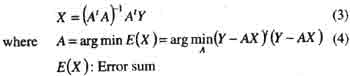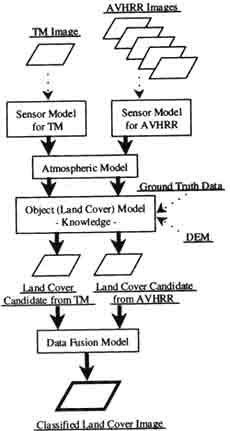Home Articles On development of land cover classification system using Remote Sensing data in...

# On development of land cover classification system using Remote Sensing data in terms of inverse problem

 ACRS 1998

Poster Session 2

On Development of Land Cover Classification system using Remote Sensing Data in terms of Inverse Problem

Junichi Suzaki and Ryosuke Shibasaki
Center for Spatial Information Science and Institute of Industrial Science
University of Tokyo
7-22-1, Roppongi, Minato-ku, Tokyo, 106-8558 Japan
Tel: +81-3-3402-6231, Fax:+81-3408-8268
E-mail: [email protected]

Abstract
Considering the increase of satellite sensors in future, the data fusion process for land cover and land use monitoring will be inevitable for the effective use of those data. In this pear, the land cover classification system is regarded as a kind of inverse problem so that it can have a certain generalization which can be flexible for the fusion of data form different kinds of sensors. Then, the framework of such a classification system is presented.

1. Introduction
Recently, land cover and land classification on a huge scale, e.g. national or continental scale, has become more and more important,. Land cover and land use data are now required to have wide coverage and relatively high accuracy . especially, extraction of crop fields, paddy fields and deforested area should be paid more attention to as a result of human impacts to the environment. Remote sensing satellite can cover wide areas efficiently, and land cover and land use classification algorithm for remotely sensed images have been studied so far. In terms of use of point-based prior information , like ground truth data, classification methods are decided into two types, supervised classification and unsupervised one. One to the most popular method among supervised classification ones in Maximum Likelihood Method. It calculates similarity (likelihood ) between the target pixel and training data set, and determine which class the pixel belongs to according to the likelihood. As a traditional unsupervised classification method, clustering is offering applied because it can produce classes, in which each pixel has some similarity based on Euclidean distance. Among them, it is common that the distance in the characteristic space is calculated and is used as criteria for the determination. In terms of use of specific information, e.g. knowledge’s on land cover characteristics , and expert system has been proposed as one of knowledge-based classification system.

Those classification methods provide estimations by using observed data and setting certain assumption against unknown parameter in many stages under some heuristic knowledge. Such a backwatds estimation problem, called as “inverse problem “, has been inevitable and the solution processes have been analyzed in various fields, e.g. geo-technical engineering, biophysics, and bio-medical engineering.

In our research, authors formulate the classification process as a kind of inverse problems,, then propose a framework, handling of knowledge information in section 2, an inverse problem approach for land cover classification using remote sensing data is described. In section 3, land cover classification system using TM and AVHRR images is explained as an example of the approach. Finally concussions are described in section 4.

2. Land Cover Classification system as a Process of Solving Inverse Problem.

2-1 Land Cover Classification System as a Process of Solving Inverse Problem
Figure 1 shows models for remote sensing process; sensor model, atmosphere model and object ( land cover ) model. Sensor model depends on each sensor type. Land cover model includes knowledge on land covers, e.g. spectral characteristics, seasonal changes, climate conditions and agricultural practices.

Simple linear regression model can be assumed for a model in Figure 1.

 Y=AX (1)

Where Y : observed data matrix
(dependent variable ) (nx1)
A: parameter matrix (nXm)
X: independent variable matrix
(mXI)

The solution approach is defined as “inverse problem ” t estimate A by using Y and X, or estimate X by using Y and A. In remote sensing field, estimation of geographical characteristics from observed data, i.e remote sensing data, is a typical inverse problem. In Figure 2, the inverse problem approach for the models in Figure 1 is shown.Figure .1 Remote Sensing Process ModelFigure.2 Inverse Problem Approach

III-posed problems are defined as the problems which cannot satisfy at least one conditions regarding the solution as below.

• existence
• uniqueness
• continuity
• continuity
• stability

 ACRS 1998

 Poster Session 2 On Development of Land Cover Classification system using Remote Sensing Data in terms of Inverse Problem The number of observed remote sensing data set is much smaller than the number of parameters , then the number of parameters, then estimation problems in remote sensing cab be dealt as ill-posed problems in inverse problem. As ill-posed problems cannot produce a unique solution specific condition is required to solve them. For example, the general solution f equation (1) is expressed as following. X=A-Y (2) Where A- : generalized inverse matrix A- has various candidates. Given the mean square error minimum condition for example, for the equation (1), the unique inverse matrix among A- and the unique solution cab be determinedAs shown above, ill-posed problems cab be solved with generalized inverse matrix and conditions, but the unique solution doesn’t always correspond with the true solution because te solution process for ill-posed problems is merely mathematical one. When dealing with geographical data in equation (1), problems such as (I) multicollinearity and (II) spatial autoregressin should be considered. Multicolinearity means that independent variables show relatively high correlating and generalized inverse matrix cannot be estimated because of reduction of A’s rank. Spatial autoregresion means that error in equation (1) shows autoregression . several strategies for the problems or regularization of ill-posed problems, are reported, and the strategies for (I) are shown s followings. (I-1) Reduction of solution space by assuming conditions (I-2) Singular value decomposition (I-3) Tikhonov regularization As for (I-3) , (3) is replaced by (5), and (4) by (6) as a result of adding he condition that norm of minimized.Assumption of conditions in (I-1) and determination of parameter c in (I-3) are dependent on empirical “knowledge ” about geographical characteristics. if such knowledge are formulated and used effectively , the never problem in remote sensing may have possibility to be solved, which leads to estimation of land cover with much higher confidence. 2-2 knowledge Handling knowledge on land cover varies form socio-economics one to ecological or geographical characteristics, e.g. agriculture practices. In some cases, there’s inconsistency between some knowledge. The processes below are thought to be necessary to involve such knowledge in the estimation system. Firstly, inconsistent knowledge should be discriminated. Second, as for inconsistent knowledge, confidence for each one should be given so that the inconsistency can be resolved in a rational manner. Then, the estimation process to unify both consistent and inconsistent knowledge should be composed. In the process, as shown in Section 2-1, certain conditions or parameters should be estimated with more accuracy or reasonability by involving such knowledge. The importance is how to express knowledge on the computers so that their inconsistency can be easily checked , and how to access the inconsistency between knowledge.Figure 3. Classified System using TM and AVHRR Images 3. Land Cover Classification System using TM and AVHRR Images Figure 3 shows a flow of classification system using TM and AVHRR images ( under development ) as an example in section 2. besides TM and AVHRR images, DEM data and ground truth data are considered as input data. And, data fusion model will function in the final part of the system to integrate both land cover candidates from TM and AVHRR more effectively. Knowledge in the object ( land cover ) model will be used opt extract some information for the inverse problem approach, s described n section 2-1. 4. Conclusions in this paper, authors formulated estimation of geographical characteristics using remote sensing as an inverse problem, on showed that the estimation process can be supported by applying knowledge on geographical characteristics. In future, considering the importance of fusion of several remote sensing data, the estimation process should have wide generalization for allowing eh fusion of different types of sensors on satellites. Reference: Kubo, S., “Inveres Problem’, Baifukan, 1992 Matsuba, Y., “A Comparative Review on Countermeasures for Patameter Estimation Problems in Regionla Modeling “, Master Thesis, Univ. of Tokyo, 1997. Bijerhammar, A., “Theory of Error and Generalized matrix Inverses “, Elsevier, 1973.